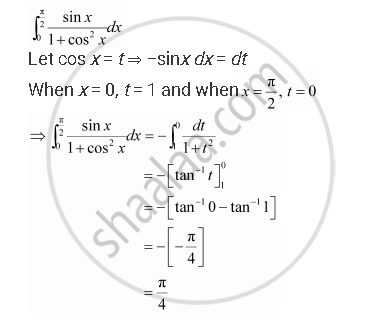Share

# Evaluate the Integrals in Using Substitution Int_0^(Pi/2) (Sin X)/(1+ Cos^2 X) Dx - CBSE (Commerce) Class 12 - Mathematics

ConceptEvaluation of Definite Integrals by Substitution

#### Question

Evaluate the integrals in using substitution

int_0^(pi/2) (sin x)/(1+ cos^2 x) dx

#### SolutionIs there an error in this question or solution?

#### Video TutorialsVIEW ALL 

Solution Evaluate the Integrals in Using Substitution Int_0^(Pi/2) (Sin X)/(1+ Cos^2 X) Dx Concept: Evaluation of Definite Integrals by Substitution.
S